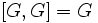# Proper and normal in quasisimple implies central

Jump to: navigation, search
This page describes additional conditions under which a subgroup property implication can be reversed, viz a weaker subgroup property, namely Normal subgroup (?), can be made to imply a stronger subgroup property, namely central subgroup
View other subgroup property implication-reversing conditions
This article gives the statement and possibly, proof, of an implication relation between two group properties. That is, it states that every group satisfying the first group property (i.e., quasisimple group) must also satisfy the second group property (i.e., group in which every proper normal subgroup is central)
View all group property implications | View all group property non-implications
Get more facts about quasisimple group|Get more facts about group in which every proper normal subgroup is central

## Statement

### Verbal statement

Any proper normal subgroup in a quasisimple group must be a central subgroup, viz it must be contained inside the center.

In other words, a quasisimple group is a group in which every proper normal subgroup is central. This is equivalent to saying that every proper subnormal subgroup is central.

## Proof

Given: A group$G$ with$[G,G] = G$ and$G/Z(G)$ simple. A normal subgroup$N$ of$G$.$Z(G)$ is the center of$G$.

To prove:$N= G$ or$N \le Z(G)$.

Proof:

1.$NZ(G)/Z(G)$ is a normal subgroup of$G/Z(G)$: Since$N$ is normal in$G$, fact (2) tells us that the image of$N$ under the quotient map$G \to G/Z(G)$ is a normal subgroup of$G/Z(G)$. This image is precisely$NZ(G)/Z(G)$.
2. Either$N \le Z(G)$ or$NZ(G) = G$: Since$G/Z(G)$ is simple,$NZ(G)/Z(G)$ is either trivial or$G/Z(G)$, so$NZ(G) = Z(G)$ (forcing$N \le Z(G)$) or$NZ(G) = G$.
3. If$NZ(G) = G$, then$G/N$ is abelian: By fact (1),$G/N = NZ(G)/N \cong Z(G)/(Z(G) \cap N)$, which is a quotient of abelian groups, hence abelian.
4. If$NZ(G) = G$, then$G = N$: Since$G/N$ is abelian,$[G,G] \le N$, so$G \le N$, since$[G,G] = G$by assumption.

Steps (2) and (4) complete the proof.